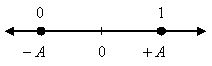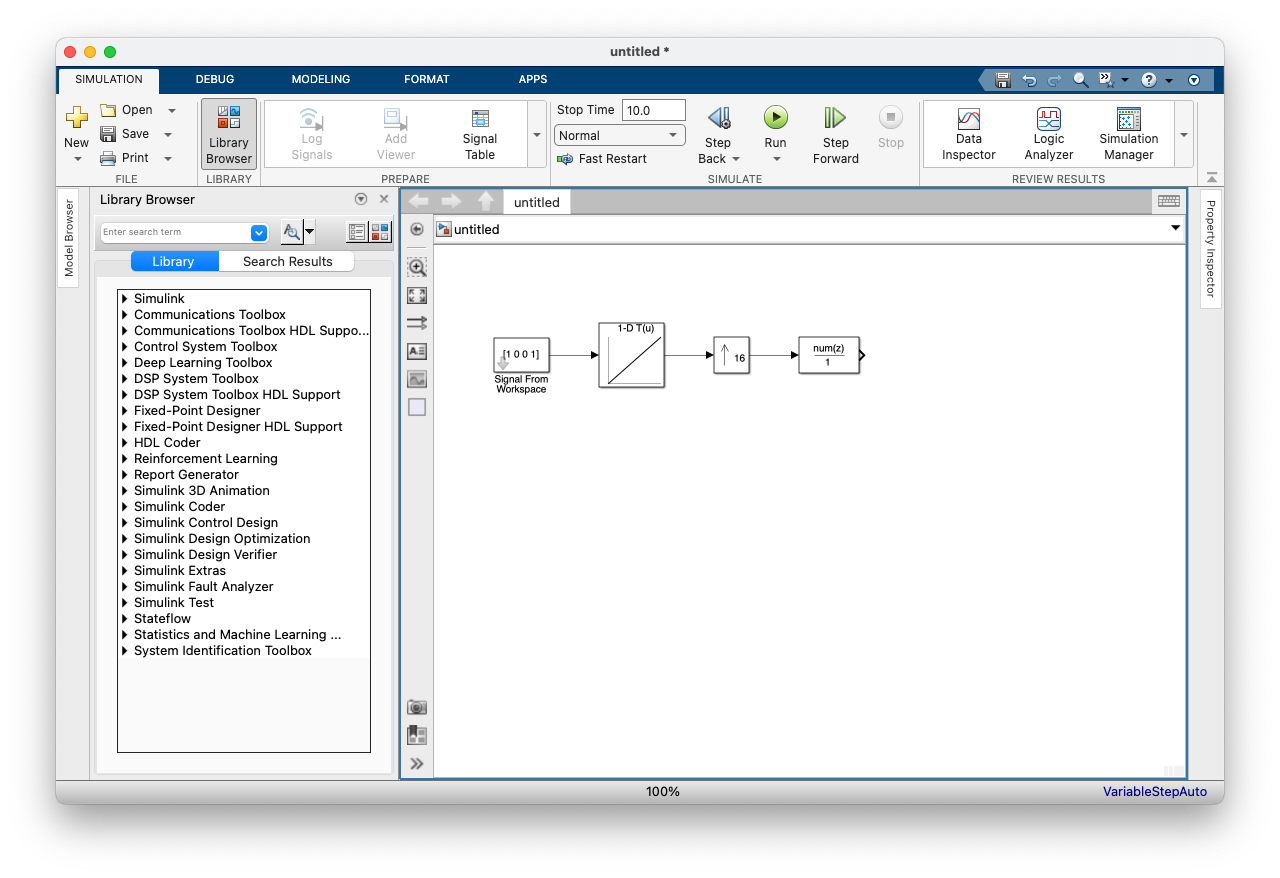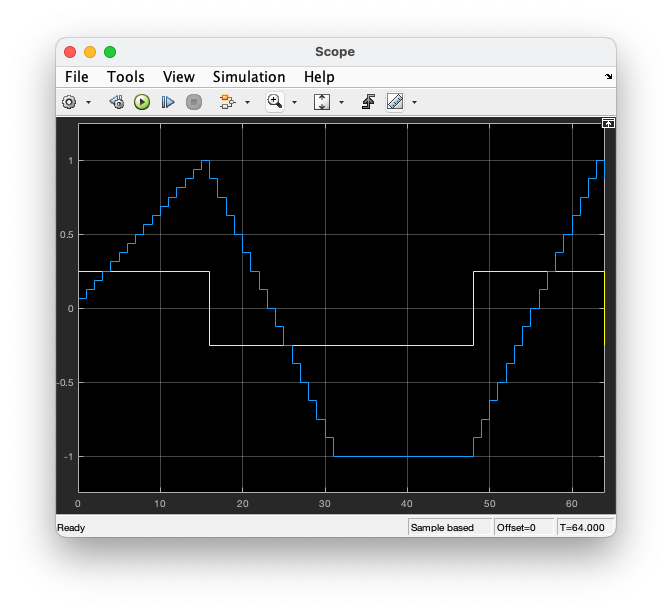# Digital Communications: A Discrete-Time Approachby Michael Rice# Binary Pulse Amplitude Modulation (PAM)

## Introduction

In this exercise, you will design a binary baseband detector to process the noisy modulated data contained in the file bb2data.mat.

## Textbook References

PAM: Section 5.2 (pp. 227 - 238), discrete-time realizations: Section 5.2.2 (pp. 233 - 238), full response pulse shapes: Section A.1 (pp. 673 - 676).

## Specificationsnormalized sample rate: 16 samples/bit average energy 1 pulse shape: NRZ symbol clock offset: 0 input file bb2data.mat input message length: 21 symbols (21 bits)

## Preliminary Design

### Design the Detector

Design the detector, shown below, using blocks from the Simulink, DSP System, and Communications System Toolboxes.### Test the Detector Design

You should test the detector you designed by constructing a modulator to produce a test signal. The following procedure steps you through this design process:
1. Design the modulator shown below to meet the above specifications except make the input the four symbol sequence 1 0 0 1.This modulator comprises four blocks
• The first block is the Signal From Workspace block:

DSP System Toolbox -> Signal Processing Sources -> Signal From Workspace

Drag this block to your design window. Double-click on the Signal From Workspace block and set the block parameters as illustrated below.The first parameter is a column vector defining the input data. The second parameter specifies the sample time. We want to normalize all sample times to the sample rate. Since there are N=16 samples/bit, 16 is enetered in this field. Since we won't be doing any frame buffering in this exercise, leave the third parameter alone. The last parameter specifies what to do when the end of the workspace vector is reached but the simulation is still running. The options are to append zeros, repeat the last value, or cycle back to the beginning of the workspace vector. It won't matter what you choose for this simulation: I just left it at the default setting as shown.

• The second block is the Look-up Table block:

Simulink -> Lookup Tables -> 1-D Look-Up Table

Drag this block to your design window. Double click on the Look-Up Table block and set the block parameters as illustrated below.The first parameter is the vector of input values which are zero and one for a binary communications system. The second parameter is the vector of corresponding output values.

• The third block is the Upsample block:

DSP System Toolbox -> Signal Operations -> Upsample

Drag this block to your design window. Double click on the Upsample block and set the block parameters as illustrated below.The first parameter is the upsample factor which is the number of samples/bit. The second parameter is an offset parameters which we don't need to use. Just leave it at the default value of zero.

• The fourth block is the Discrete Filter block:

Simulink -> Discrete -> Discrete Filter

The filter block is used to perform pulse shaping. Drag this block to your design window. Double click on the Discrete Filter block and set the block parameters as illustrated below.2. Connect the output of your modulator to the input of your detector.

3. Connect the output of your detector to a To Workspace block. Be sure to open the Properties Dialog Window and set the Save format to matrix.

4. Set the simulation parameters as follows:
 Simulation Time
 Start Time: 0.0 Stop Time: 4*16
Solver Options
 Type: Fixed-step Solver: discrete (no continuous states) Fixed step size: auto
 Periodic sample time constraint: Unconstrained Tasking mode for periodic sample times: SingleTasking

5. Run the simulation and plot the demodulator input and the matched filter output on the same set of axes. You should get a plot that looks like this.The solid yellow line is the received signal (the test signal) and the dashed line is the matched filter output. As you can see, the first valid bit decision occurs at sample 16 which corresponds to the first peak observed in the matched filter output. The three subsequent bit decisions at samples 32, 48, and 64 correspond to the next three bits in the data sequence. If you look in the workspace, you will notice that the detector output 5 decisions instead of 4. This is because the Downsample block output an initial 0 at sample 0.

6. Does the workspace variable agree with the input sequence (1 0 0 1)? Make sure the "phase" of the Downsample block is set properly so that the right matched filter output sample is the one that is kept. You'll have to experiment with this you want +1 or -1 at the output of the matched filter. You may need to run the simulation to stop time of 5*16 depending on the offset you use. When you debug your system so that it does, you are ready to work on the exercise.

## Exercise

1. Replace the modulator blocks with the From File block and set the Filename to bb2data.mat and the sample time to 1.

2. Set the simulation parameters as follows:
 Simulation Time
 Start Time: 0.0 Stop Time: 16*(3*7)
Solver Options
 Type: Fixed-step Solver: discrete (no continuous states) Fixed step size: auto